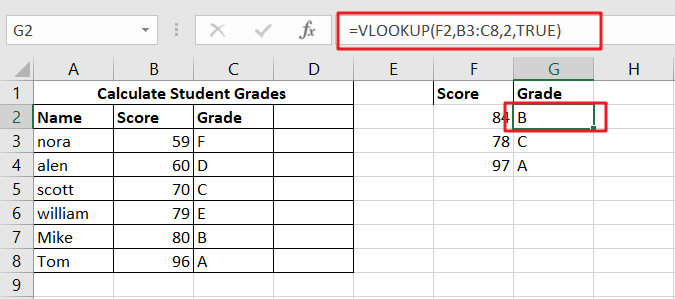# Calculate Grades With VLookup in Excel

## Why Should You Calculate Grades With VLookup Excel?

If you’re looking for a simple way to find out a student’s grades, VLOOKUP Excel can do the trick. This function uses a lookup table to find the values and sort them in ascending order. It also requires the values to be in ascending order. The table itself should be named in the same way as the grades.

VLookup has the ability to adjust grade points for natural breaks in the distribution. This way, you’ll be able to analyze how a student performs on an assignment. Then, you can adjust the grade points and the grade point table. If you’re unsure how to use VLookup Excel, just check out the steps below!

## Calculate Grades With VLOOKUP ExcelIf you’re trying to figure out how to calculate grades with VLOOKUP Excel, you’re in the right place. The VLOOKUP function can be used in a variety of ways, from calculating averages to performing a lookup on results. It can also be used to determine a student’s grade, which is particularly useful for educational institutions. However, before you can use VLOOKUP Excel to determine a student’s grade, you need first to figure out the scale of their results.

When you use the VLOOKUP Function in Excel, you’ll need a table with column values sorted in ascending order. Then, you need to set up an “approximate match” condition. The VLOOKUP function works by searching through a table for data. It looks up the value in a column based on its row or column index. The lookup value will return an approximate match when it meets the input value.

### Generic Formula

`=VLOOKUP(score,key,2,TRUE)`

### Syntax And Explanation

`=VLOOKUP(E5,key,2,TRUE)`By putting in the values from the above-given screenshot, we can easily calculate grades using the Vlookup function!

### Summary

VLOOKUP is an Excel function that will look up values from another table and return the value in the same cell. In this example, the result is the grade of student X. The formula can be pasted in any cell, even next to other students. If the formula has too many variables, you can use the Fill Down function from the Edit menu. The VLOOKUP function is easy to use and will save you a lot of time.

## Conclusion

If you’re wondering whether you should use VLOOKUP for calculating grades, you may want to read this article. It will show you the advantages of using the function to make this type of calculation. It will also help you find grades for all students using the same criteria, such as name or exam level. This type of formula will update automatically with any changes to the table. To use VLOOKUP, you’ll need to set up a table with scores in it. You can then sort the data by column and enter a formula that will assign each grade.

### Related Functions

• Excel VLOOKUP function
The Excel VLOOKUP function lookup a value in the first column of the table and return the value in the same row based on index_num position.The syntax of the VLOOKUP function is as below:= VLOOKUP (lookup_value, table_array, column_index_num,[range_lookup])….